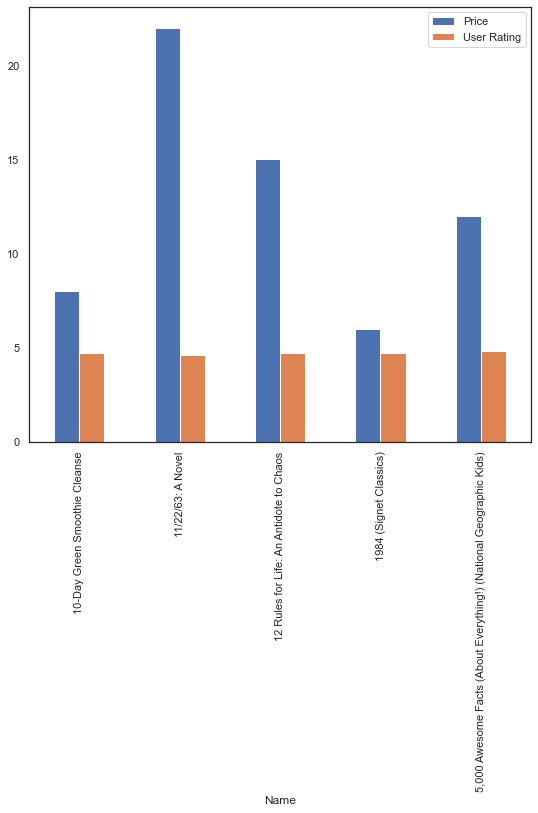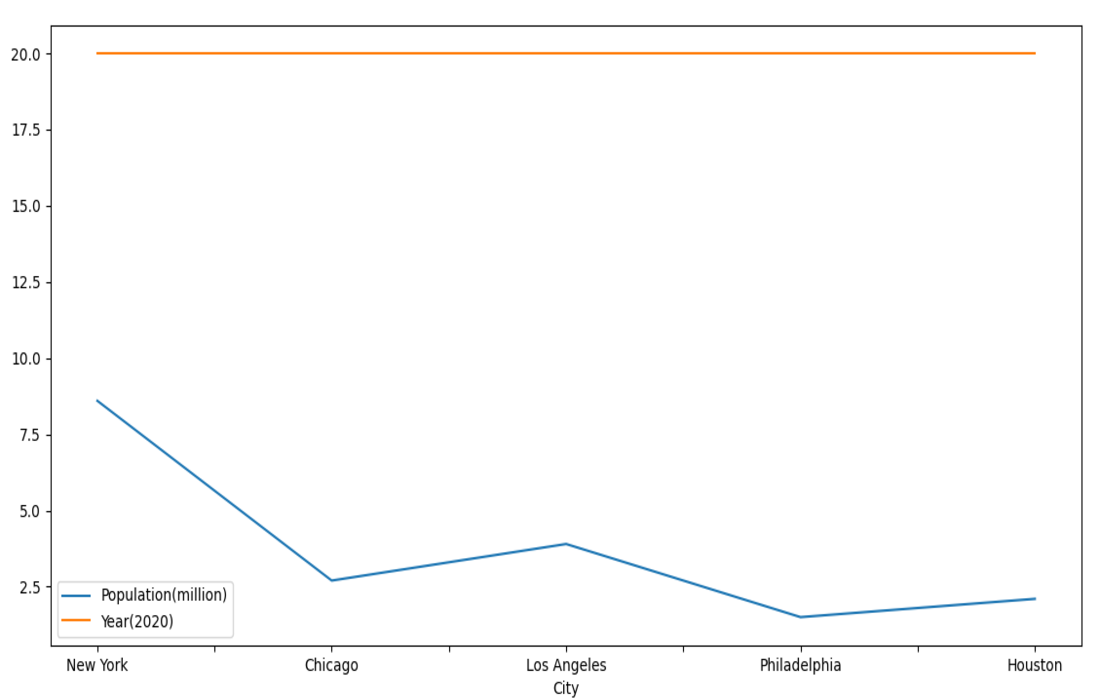Related Articles
How to plot multiple data columns in a DataFrame?
• Last Updated : 23 Feb, 2021

Prerequisites: Pandas

Python comes with a lot of useful packages such as pandas, matplotlib, numpy etc. To use dataframe, we need pandas library and to plot columns of a dataframe, we require matplotlib. Pandas has a tight integeration with Matplotlib. You can plot data directly from your DataFrame using the plot() method.

To plot multiple data columns in single frame we simply have to pass the list of columns to the y argument of the plot function. Given below is aproper approach to do so along with example implementation.

### Approach:

• Import module
• Convert to dataframe
• Using plot() method, specify a single column along X-axis and multiple columns as an array along Y-axis.
• Display graph.

Below are few examples which illustrates the above approach to plot multiples data columns in a Dataframe.

Example 1:

Database: Bestsellers

## Python3

 `import` `pandas as pd``import` `matplotlib.pyplot as mp`` ` `# take data``data ``=` `pd.read_csv(``"Bestsellers.csv"``)`` ` `# form dataframe``data ``=` `data.head()`` ` `df ``=` `pd.DataFrame(data, columns``=``[``"Name"``, ``"Price"``, ``"User Rating"``])`` ` `# plot the dataframe``df.plot(x``=``"Name"``, y``=``[``"Price"``, ``"User Rating"``], kind``=``"bar"``, figsize``=``(``9``, ``8``))`` ` `# print bar graph``mp.show()`

Output:Example 2:

## Python3

 `import` `pandas as pd``import` `matplotlib.pyplot as mp`` ` `# data to be plotted``data ``=` `[[``"New York"``, ``8.6``, ``20``],``        ``[``"Chicago"``, ``2.7``, ``20``],``        ``[``"Los Angeles"``, ``3.9``, ``20``],``        ``[``"Philadelphia"``, ``1.5``, ``20``],``        ``[``"Houston"``, ``2.1``, ``20``]]`` ` `# form dataframe from data``df ``=` `pd.DataFrame(data, columns``=``[``"City"``, ``"Population(million)"``, ``"Year(2020)"``])`` ` `# plot multiple columns such as population and year from dataframe``df.plot(x``=``"City"``, y``=``[``"Population(million)"``, ``"Year(2020)"``],``        ``kind``=``"line"``, figsize``=``(``10``, ``10``))`` ` `# display plot``mp.show()`

Output:Attention geek! Strengthen your foundations with the Python Programming Foundation Course and learn the basics.

To begin with, your interview preparations Enhance your Data Structures concepts with the Python DS Course. And to begin with your Machine Learning Journey, join the Machine Learning – Basic Level Course

My Personal Notes arrow_drop_up